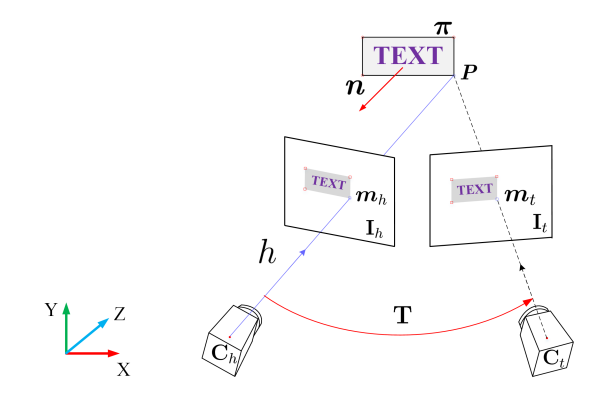# 1. 线特征

Tip：（对于向量(x,y,1)，可以在几何意义上理解为是在【第三维为常数的】平面上的一个二维向量。这种用【三】维向量表示【二】维向量，或者一般而言，用一个n+1维的向量表示一个n维向量的方法称为齐次坐标表示法。】）。

# 2. 面特征

\begin{aligned} \mathbf{n} \cdot \mathbf{xx_0} &= \mathbf{n}^T \mathbf{xx_0} \\ &= a(x - x_0) + b(y - y_0) + c(z - z_0) \\ &= ax + by + cz + (-ax_0 - ay_0 - az_0)\\ &= 0 \\ \end{aligned}

$\mathbf{p} = [x_1, y_1, z_1]^T$ 到平面内的距离(D)由相关证明可得到：

$D = \frac{ax_1 + by_1 + cz_1 + d}{\sqrt{a^2 + b^2 + c^2}}$

$D = \mathbf{n}^T p + d$由homography transformation $\mathbf{H} \sim \mathbf{R}+t \boldsymbol{\theta}^{\mathrm{T}}$ ,我们可以根据该平面方程和特征点计算出整个的重投影误差 $\begin{array}{l} u^{\prime}=\left(\boldsymbol{r}_{1} \tilde{\boldsymbol{m}}+t_{1} \tilde{\boldsymbol{m}}^{\mathrm{T} \boldsymbol{\theta}}\right) /\left(\boldsymbol{r}_{3} \tilde{\boldsymbol{m}}+t_{3} \tilde{\boldsymbol{m}}^{\mathrm{T}} \boldsymbol{\theta}\right) \\ \boldsymbol{v}^{\prime}=\left(\boldsymbol{r}_{2} \tilde{\boldsymbol{m}}+t_{2} \tilde{\boldsymbol{m}}^{\mathrm{T}} \boldsymbol{\theta}\right) /\left(\boldsymbol{r}_{3} \tilde{\boldsymbol{m}}+t_{3} \tilde{\boldsymbol{m}}^{\mathrm{T}} \boldsymbol{\theta}\right) \end{array}$

$m^{\prime}=h\left(m, T_{h}, T_{t}, \theta\right)$

# 3. 参考链接

https://zhuanlan.zhihu.com/p/41316938

https://scm_mos.gitlab.io/slam/text_feature/